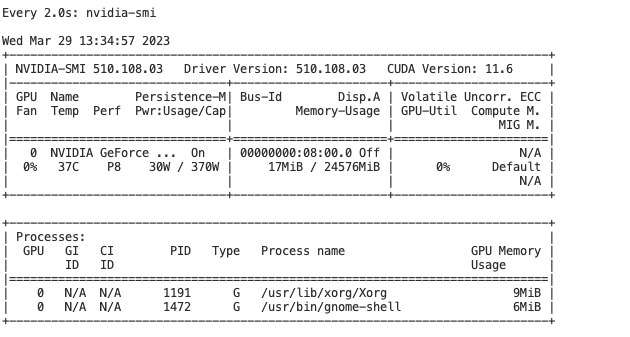# Cuda out of memeory

I am using the following code to reconstruct 3D volume from the 2D segmented slices- which I am getting from my 2D model. I donot want to calculate loss here. My batch size is 4, the slice dimension that is being passed to 2D model is [1,1,256,256] (one image at a time). However, given the slice dimension, I am still getting the cuda out of memory error. `torch.cuda.OutOfMemoryError: CUDA out of memory. Tried to allocate 20.00 MiB (GPU 0; 23.70 GiB total capacity; 5.36 GiB already allocated; 13.00 MiB free; 5.37 GiB reserved in total by PyTorch) If reserved memory is >> allocated memory try setting max_split_size_mb to avoid fragmentation. See documentation for Memory Management and PYTORCH_CUDA_ALLOC_CONF`

``````for epoch in range(1, num_epochs + 1):

model.train()
train_dice_total = 0.0
num_steps = 0

print(len(batch))

input_samples, gt_samples, voxel_dim = batch
input_samples = input_samples.float()

if torch.cuda.is_available():
input_samples = input_samples.cuda(device= "cuda")
var_gt = gt_samples.cuda(device = "cuda")
model = model.cuda()

# Initialize an empty tensor to store the segmented volume
segmented_volume = torch.zeros((input_samples.shape))

# Iterate over each image in the batch
for img_id in range(input_samples.shape):
# Get the slices for the current image
img_slices = input_samples[img_id]

# Initialize an empty tensor to store the segmented slices for the current image
segmented_img_slices = torch.zeros((img_slices.shape))

# Iterate over each slice in the current image
for slice_id in range(img_slices.shape):
# Get the current slice
slice = img_slices[slice_id]

# Add a batch dimension to the current slice
slice = slice.unsqueeze(0)
print("slice", slice.shape)
# Pass the current slice through the model to get the segmentation mask
segmented_slice = model(slice)

# Remove the batch dimension from the segmented slice
segmented_slice = segmented_slice.squeeze(0)

# Add the segmented slice to the list of segmented slices for the current image
segmented_img_slices[slice_id] = segmented_slice
# embed()
# Combine the segmented slices for the current image into a 3D volume
segmented_images_i = segmented_img_slices.permute(1, 0, 2, 3).unsqueeze(0)

# Add the segmented volume for the current image to the list of segmented volumes
segmented_volume[img_id] = segmented_images_i

# Remove the batch dimension from the segmented volume
segmented_volume = segmented_volume.squeeze(1)
``````

How can I resolve this error?

Based on the error message it seems other processes might use the majority of the device memory as the allocated and reserved memory is ~5.3GB while only 13MB are free. Check `nvidia-smi` to see how much memory other processes are using and close them if possible.

@ptrblck , I figure out that the process in actually getting killed in the `get_item()`. It loads 3 images only and it crashes then. I checked the memory usage with `watch nvidia-smi`, but there is no other active process. Below is its screenshot:Below is the code of my data_set class:

``````class cc359(Dataset):
def __init__(self, config, train = True, rotate=True, scale=True ):
self.rotate = rotate
self.scale = scale
self.fold = config.fold
self.train = train
self.site = config.site
self.data_path = config.data_path
self.source = config.source

if self.site == 1:
self.folder = 'GE_15'
elif self.site == 2:
self.folder = 'GE_3'
elif self.site == 3:
self.folder = 'Philips_15'
elif self.site == 4:
self.folder = 'Philips_3'
elif self.site == 5:
self.folder = 'Siemens_15'
elif self.site == 6:
self.folder = 'Siemens_3'
else:
self.folder = 'GE_3'

s, h, w = img.shape
if h < w:
b = (w - h) // 2
a = w - (b + h)
return np.pad(img, ((0, 0), (b, a), (0, 0)), mode='edge')
elif w < h:
b = (h - w) // 2
a = h - (b + w)
return np.pad(img, ((0, 0), (0, 0), (b, a)), mode='edge')
else:
return img

current_size = data_array.shape[-1]
b = (max_size - current_size) // 2
a = max_size - (b + current_size)
return np.pad(data_array, ((0, 0), (b, a), (b, a)), mode='edge')

def unify_sizes(self, input_images, input_labels):
sizes = np.zeros(len(input_images), np.int)
for i in range(len(input_images)):
sizes[i] = input_images[i].shape[-1]
max_size = np.max(sizes)
for i in range(len(input_images)):
if sizes[i] != max_size:
return input_images, input_labels

def img_transform(self, img_slice):

self.sagittal = True
scaler = None
if self.scale:
scaler = MinMaxScaler()

if self.scale:
transformed = scaler.fit_transform(np.reshape(img_slice, (-1, 1)))
img_slice = np.reshape(transformed, img_slice.shape)

if not self.sagittal:
img_slice = np.moveaxis(img_slice, -1, 0)

if self.rotate:
img_slice = np.rot90(img_slice, axes=(1, 2))
if img_slice.shape != img_slice.shape:

return img_slice

images = []
labels = []
self.voxel_dim = []
img_slice_id = []
gt_slice_id = []

if self.source and self.train:
images_path = os.path.join(data_path, 'Original', self.folder, "train")
print("image_path ", images_path )
elif self.source and not self.train:
images_path = os.path.join(data_path, 'Original', self.folder, "val")
print("image_path ", images_path )
else:
print(self.source, self.train)
images_path = os.path.join(data_path, 'Original', self.folder)
print("image_path ", images_path )

files = np.array(sorted(os.listdir(images_path)))

for i, f in enumerate(files[:4]):
print("correct_file", f)

self.voxel_dim.append(np.array(spacing))

#             loop over slices and save each slice with its corresponding file name and slice ID
for slice_id, img_slice in enumerate(nib_file.get_fdata('unchanged', dtype=np.float32)):

img_slice = np.expand_dims(img_slice, axis=0)  # add channel dimension
img_slice = cc359.img_transform(self, img_slice)
images.append(img_slice)
img_slice_id.append(f'{f[:-7]}_id_{slice_id}')

lbl = nib.load(os.path.join(data_path, 'Silver-standard', self.folder, f[:-7] + '_ss.nii.gz')).get_fdata(
'unchanged', dtype=np.float32)
img_slice = cc359.img_transform(self, lbl)

labels.append(img_slice)
#                 gt_slice_id.append(f'{f[:-7]}_ss_id_{slice_id}')

images, labels = self.unify_sizes(images, labels)
self.data = torch.from_numpy(np.expand_dims(np.vstack(images), axis=1))
self.label = torch.from_numpy(np.expand_dims(np.vstack(labels), axis=1))
self.voxel_dim = torch.from_numpy(np.vstack(self.voxel_dim))
self.img_file_names = np.array(img_slice_id)
#         self.gt_file_names  = np.array(gt_slice_id)

#         print("self.data", self.data.shape, "self.label:", self.label.shape,
#               "self.voxel_dim", self.voxel_dim.shape, "len", len(self.voxel_dim.shape))

def __len__(self):
return len(self.data)

def __getitem__(self, idx):

data = self.data[idx]
label = self.label[idx]
voxel_dim = self.voxel_dim[idx]
img_file_names = self.img_file_names[idx]
#         gt_file_names = self.gt_file_names[idx]

return data, label, voxel_dim, img_file_names
``````

I also ran dmesg, which gave me the following error:

``````[ 2062.610388] Out of memory: Killed process 9424 (python) total-vm:86435024kB, anon-rss:63197844kB, file-rss:0kB, │
You are running out of host memory based on the `dmesg` output and should check how large each sample is and why the process uses so much RAM.